American Journal of Optics and Photonics
Volume 3, Issue 5, October 2015, Pages: 89-93

Quanto-Optical Effects of Exciton-Polariton System

Arezu Jahanshir

Department of Eng. Physic, Buein Zahra Technical University, Qazvin, Iran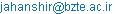Arezu Jahanshir. Quanto-Optical Effects of Exciton-Polariton System.American Journal of Optics and Photonics.Vol.3, No. 5, 2015, pp. 89-93. doi: 10.11648/j.ajop.20150305.16

Abstract: The mutual quantum and optical effects of exciton bound states in exciton-polartiton system in the inhomogeneous multi-layer environment in semiconductor quantum dots (SQDs) are presented. We theoretically show that how optical effects (polarization) of exciton-polariton system can affect on quantum characteristics (spin projections) which results in beats between right- and left-circularly polarized photoluminescence. Optical polarization effects in exciton-polariton interactions in SQDs enable complete studies of the exciton-polariton spin dynamics. The theoretical information on the exotic-polariton states and definition of Hamiltonian interactions according to the basis investigation of the asymptotically bihaviour of the loop function of scalar particles (electron-hole) in SQDs are determined. Using oscillator representation method, spin Hamiltonian coefficient of excitons with effective confinement potential, Coulomb and spin effects are determined. In addition we can say that controlling and understanding of spin interaction between electron- holes localized in SQDs can controlled optically effects and interactions. Spin quantum manipulations can be useful in new generation of optic-photonic studies, especially super quantum computers, Bose condensates of exciton-polariton, polariton lasers and etc.

Keywords: Exciton-Polartiton, Spin Interaction, Optical Polarization, Multiple Spin Projections

Contents

1. Introduction

The interaction of light and exotic bound state system (exciton) in semiconductors has recently become of great interest because of the additional spin-spin interactions allowed [1,2]. Originating of photons with exciton bound states (electron-hole pairs) produce mixed quasi-particle systems called exciton-polariton with new and remarkable properties. In particular, interactions between the electron-hole, exciton-exciton and exciton-polariton can be important for new surveying quanto-optical effects which could be useful in modern technology. The parametric scattering and interactions of pairs of electronic quasi-particles can be described either through QFT, QED and nonlinear physics, but in this article the exciton- polariton considered through QFT and QED. Here spin interactions of ground and excited state of exciton-polariton systems will be studied. The exciton-polariton has spins interactions, characteristics and Hamiltonian of interactions based on Coulomb potential should be surveyed [6,7] by QFT and QED. At the present time the technical achievements of experimental studies make it possible to obtain the SQDs characteristic better, so determination of these new characteristics should be based on quantum and relativistic effects in the electromagnetic field. We will define these parameters, according to the asymptotic bihaviour of the loop function in the scalar electrodynamics field and use the oscillator representation method (ORM) . The ORM based on the free field representation of Hamiltonian for the system. This Method supports us to have useful descriptions for the unification of particle and quasi-particles bound states inside the SQDs by using QFT and QED theories. Therefore here, we worked on spin effects in Nano-crystals constructed by exciton-polariton system in the SQDs. ORM has been used to show that how spin Hamiltonian constant coefficients of excitons in exciton-polariton system will be important in modern technology and new quantum based equipment like super quantum computers.

2. Exciton-Polariton System

The interaction mechanism and quasi- particle properties are well described in the framework of QFT , which describe quantum properties of such objects. The elementary particles and quasi-particles are quantum objects because they possess a wave and corpuscular character. Therefore the interaction of light (photon/cavity photon) and exotic electron-hole bound state system (exciton) in semiconductors have recently become of great interest because of the additional spin-spin interactions allowed [5,7]. This exotic quasi-system named after exciton-polariton system, which is a bosonic quasi-particle that exists inside SQDs, consisting of an exciton and a cavity photon. As this quasi-system of exciton-polariton exists of electrons, hole and photons, and cavity photon therefore quantum characteristic like spin-orbital and spin-spin interactions, are a central subject in the emerging QFT and QED theories to describe new formation of matter which we know as exciton-polariton condensates matter and will be new area of modern and high quantum technologies that is very important in Cavity Quantum electrodynamics. Since the qubit (spin of a single electron in SQDs) [5-7]is a main description for the realization of scalable quantum computers and new generation of super quantum computers, so spin interaction in exciton bound states will be important in exotic quasi-system of exciton-polarition. In this article a method, based on bound states and QFT conditions, is developed for determination and calculation of the quanto-optical properties of exciton and exciton- polariton systems with spin interactions. The method in a general manner for an optional electron-hole and electron-hole-photon potential and in the more specifically approach, based on exciton bound state conditions is formulated. Last approach yields analytical results for exotic and quasi-exotics system in SQDs and it allows us to analyze correlation between quantum and optical effects of the exciton. In this paper we determine a part of quanto-optical effects (spins interaction) between exotic systems (exciton-polariton). Therefore the spin-orbit interactions in the holes (valence-band) are the principal spin studies mechanism in SQDs.

3. Exciton Spins Projection

Single electron spins, hole spins and excitons in the single state due to quantum confinement can dominant heavy-hole and light-hole character which have difference in effective mass of main particles [9-12]. In SQDs a particle's effective mass is the mass that it seems to have when responding to forces or the mass that it seems to have when in group with other identical particles in physical states. For electron/hole in SQDs, the effective mass is usually stated in units of the true mass of the electron and it is usually in the range 0.01 to 10, but can also be lower or higher in heavy hole and light hole. In exotic system heavy hole effective mass reaches 1,000. Therefore for the electronic effective mass can be seen as an important basic parameter that influences measurable properties of SQDs that is directly include in spintronics effects in SQDs. The light-hole and the heavy-hole exciton, which are a quasi-particle formed from a single electron bound to a single light-hole or heavy- hole, are very important for quantum information science and spintronics technology applications. But the quanto-optical effects [13,15] of exciton-polariton dynamics system with light-hole in SQDs which including the exciton-exciton and exciton-phonon interaction are very different to the more commonly known heavy-hole exciton. In a semiconductor structure the light-hole has a spin projection ±1/2, heavy-hole has a spin projection ±3/2 and electron has a spin projections: ±1/2. Therefore based on these spin projection and especial quantum structure of semiconductors exotic bound states system will be get different spin-spin and spin-orbital interactions with photon, electron and hole [12-16]. So using of equations (3) and (4) will be important to describe Hamiltonian and spins interactions in semiconductors. Interactions results between electron, hole and quasi-particle bound states will be involved with quanto-optical effects i.e. left circular polarization (LCP) and right circular polarization (RCP)characteristics. For example based on polarization characteristics we get dark exciton or exciton-polariton systems with heavy-hole which has spins interaction as follow:

Table 1. Quanto-optical transitions of light and heavy holes.

 Heavy-hole spin projection +3/2 -3/2 Electron spin projection +1/2 +2 (Dark exciton) -1 (Bright exciton) Exciton-polariton (RCP) -1/2 +1 (Bright exciton) Exciton-polariton (LCP) -2 (Dark exciton) Light-hole spin projection +1/2 -1/2 Electron spin projection +1/2 +1 Exciton-polariton 0 Exciton-polariton -1/2 0 Exciton-polariton -1 Exciton-polariton

4. Spintronics Coefficients in SQDs

The development of the quantum information concept has resulted in the creation of spintronics or spin electronics which describes the spin’s role of electron or exotic particles in solid state physics, and possible devices that specifically exploit spin properties. Spintronics is an emerging field of nanoscale electronics involving the detection and manipulation of electron spin. On the other words spintronics technology use electron-exotic particles spins to build faster and more efficient devices. Thus demonstration of electrons, particles and quasi-particles interactions will be based on spin-orbital quantum characteristics. According to Spintronics theories effective parameters in interactions of particles can be determined only within the framework of QFT and QED. In this case we will describe interactions and particles characteristics in the external electromagnetics filed. The main structure in SQDs is exciton bound states. A fundamental problem in a description of the mechanism of coupled state interaction in spin approaches is the determination of spin-orbital interactions. At the first moment we try to describe interaction of two charged scalar particles in an external gauge field and assume that the system of these particles forms a coupled state. We now determine and define the spin-orbital effects based on the asymptotical behavior of the polarization closed loop function for the charged scalar and averaging over the gauge field, and also definition of the Green function for scalar particles in an external gauge field based on total Hamiltonian. Total Hamiltonian of interaction in inhomogeneous environment of SQDs is explained through electrostatic forces, electron relative potential (resulted from dissemination of charge and electron flow, which result to formation of related electromagnetic field between electrons and electrons-holes, spin effects and particles’ complementary effects. Therefore to determine the spin-orbital interaction the eigenvalue of the total Hamiltonian must be known so the interaction Hamiltonian must be specified with allowance for spin-orbital interactions [1, 18, 19]. Schrödinger equation for exciton system is sum of Hamilton’s effect of potential field (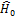), complementary Hamilton’s effect (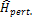), spin-orbital Hamilton’s effects (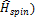given as: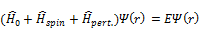(1)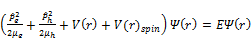(2)

In the exciton system Hamilton’s equation formed by different loads with Coulomb force equal to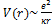[20, 21]. All Hamilton of particles should be determined through surveying spin-spin and spin-orbital effect between particles in quantum dots. In this area, force between particles is Coulomb electrostatic potential, the force which is result of holes effects, quadrupole forces and the force resulted from electron –hole relative movement. We have only considered the potential resulted from first two forces. So final potential in quantum dots will be equal to vector potential resulted from uni-photon transaction and the potential resulted from electrons’ confinement and spin effect [22-4]. Election of distance between electron and hole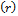in exciton system for calculating spin-spin and spin-orbital effects will be so that total vector potential effect resulted from uni-photon exchange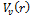have overlap with the potential resulted from electron converge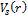: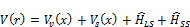(3)

Therefore, relations of quantum numbers with spin-orbital effects’ operators for two particles can be determined by: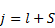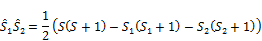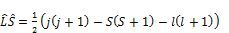(4)

Nowadays, calculating energy spectrum with coulomb potential and considering relativistic features of bound state system has attracted many researchers. This paper aims at using oscillator representation method of total Hamiltonian of exciton-polariton. We determine characterizes of a bound state in asymptotical behavior of the polarization loop function for two scalar particles in external electromagnetic field. The polarization operator in an external electromagnetic field looks like [27-28]: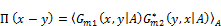(5)

Here is taking the average on external statistical field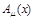. The Green function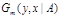of a scalar particle in external gauge field looks like: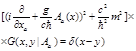(6)

where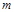- mass of a scalar particle, and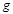- is the coupling constant of interaction. In this case, we obtain the non-relativistic limit with of the loop function. The gauge field averaging is defined as follows: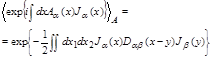(7)

Here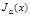is a real current, and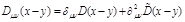(8)

The solution of (2) can be represented in the form of the following functional integral: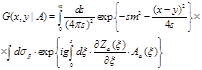(9)

Where we used the notations: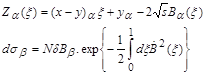The conditions: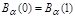and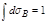Substituting (9) in (5) and after some calculations, we get for the loop function: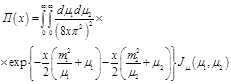(10)

Where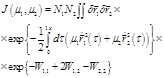(11)

and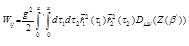(12)

The functional integral in (9) is similar to the Feynman Path integral trajectories in non-relativistic quantum mechanics  for the motion of two particles with masses. The interaction of these particles is described by the nonlocal functional in (12), in which are contained both potential and non-potential interaction. Therefore, we must determine the potential structure of the Hamiltonian interactions, and then the eigenvalue of the total Hamiltonian which is sum of potential, spin-spin, spin-orbital and nonperturbative interactions. For this purpose, we consider following Schrödinger equation [21,23]: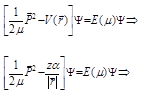(13)

where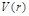is the Coulomb interaction potential and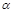is the coupling constant of electromagnetic interaction. From this equation we find the eigenvalue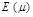for total Hamiltonian interactions which contain spins interactions. We will use this definition to describe exciton-polariton spins effects in spintronics technology theories. As we know spins equations formed by electron and hole, which suppose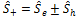for sum of two particles’ spins in exciton could be determined by separated parts of spin-orbital and spin-spin equations as follow: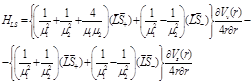(14)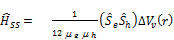(15)

Thus, Hamiltonian of spins interaction will be: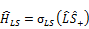and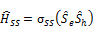Where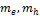are mass of electron and hold,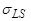is spin-orbit and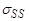is spin-spin Hamilton constant coefficient. Now using oscillating method in spherical coordination and changing parameters in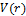, parameters related to particles’ distances in exciton system for electrostatic coulomb potential ruling in SQDs s and overlap of spin potential would be calculated. As we know, exciton radius will be calculable through separating sections related to constituent mass of exciton system, effective mass of electron/hold, mass center and relative movement through Wannier Equation. These equations clearly determine that there is a direct relation between spin Hamiltonian constant coefficient with visual load sum near to layers’ border and an indirect relation between system reduced mass and exciton radius. By spintronics ideas and theories, we are working on spins effects in Nano-crystals constructed by multi-exciton, and multi exciton-polariton systems in space between SQDs. In this case oscillating calculation method has been used to calculate spins effects.

5. Conclusion

The result of the electrostatic Coulomb interaction between electrons, holes, photons which becomes spin-dependent because of the requirement that the wave function of a pair of electrons be anti-symmetric with respect to the interchange of electron coordinates and spins, we could be describe t new characteristics of quanto-orbital effects in all type of semiconductors like quantum dots, quantum well and etc. An exciton in a semiconductor is again a hydrogen-like system with properties that can move inside the semiconductor. Usually they are created by optical excitation. Thus the spin–orbit interaction can be important characteristic that depends not only on the velocity of the electron-hole, but also on the structure of crystal when interact not directly with electromagnetic light (photon). More details can be found in spin–orbit a Hamiltonian interaction which is the key to this subject and it makes the transport and spin phenomena inter-dependent in spintronics technology. Therefore based on theoretical description, we can serve to enumerate the possible types of spin interactions that can be encountered in a semiconductor. Thus, based on spin projection of components in semiconductors which means that the probability that two particles are very close to each other is small compared to the opposite case, when the spins are antiparallel we can get different optical effects in systems. Therefor we introduce with two different type of exotic exciton bound states and exotic quasi system like exciton-polariton systems. Therefore, to describe exact characteristics of semiconductor which is very important in spintronics technology, determination and definition of spins Hamilton’s are the main part of theoretical calculations. Thus in this article we tried to introduce readers with the oscillator representation method which is good way to determine spins interactions based on QFT and QED theories.

References

1. Yu. D. Zavorotnev and O. Yu. Popova, "Polariton Spectrum Subject to Bose-Einstein Condensate of Excitons’, Journal of Photonic Materials and Technology; 1(1), 10-14, 2015.
2. V. V. Rumyantsev and  K. V. Gumennyk, "Towards a History of Concepts of Light-Matter Coupling ", Journal of Photonic Materials and Technology, 1(1), 1-9,  2015.
3. M. Dineykhan, G.V. Efimov and Kh. Narmsrai, "Investigation of Green Functions and the Parisi-Wu Quantization Method in Background Stochas­tic Fields", Fortsh. Phys., 39, 259-318, 1999.
4. R.P. Feynman, A. P. Hibbs, "Quantum Mechanics and Path Integrals", New York McGraw-Hill, 1965.
5. M. Dineykhan, A. Jahanshhir, et al., "Mass spectrum bound state system with relativistic corrections", Journal of physics B: Atomic, Molecular and Optical Physics, 42(1), 145001, 2009.
6. M. Dineykhan, A. Jahanshir, et al., "Effective Hamiltonian in scaler electrodynamics", Preprint, 197(p2-2002-197). Dubna, Russian, 1-20, 2002.
7. M. Dineykhan, G.V. Efimov, "The Schrodinger equation for the Bound State Systems in the Oscillator Representation", Reports on Mathematical Physics, 36, 287-307, 1995.
8. M. Dineykhan, R.G. Nazmitdinov, "On analytical solutions to the problem of the Coulomb and confining potentials", Yad. Fiz., 62, 143-148, 1999.
9. A. Jahanshir, "Exotic pi-atom", First ICP, Iran, 679-682, 2004.
10. M. Dineykhan, G.V. Efimov, G. Ganbol, "Oscillator rep­resentation in quantum physics", Springer- Verlag, Berlin, 1995.
11. A. Jahanshir, "Mesonic hydrogen mass spectrum in the oscillator representation", Journal of Theoretical and Applied Physics, 3(4), 14-17, 2010.
12. W. Lucha, F. Schoberl, D. Gromes," Bound states of quarks", Phys. Rep. 200, 127-135, 1991.
13. W.Greiner and Y.Reinhort, Q"uantum Electrodynamic", 1st edition, Springer- Verleg, 1992.
14. A. Jahanshir, "Spin-Orbital Effect of Diexciton in Quantum Dots", American Journal of Condensed Matter Physics, 2(3), 57-62, 2012.
15. A. Jahanshir, "Hamiltonian Multiplex Interaction Based on Excitons Effect in Semiconductor QCs", Scientific Journal of Pure and Applied Sciences, 3(11), 878-880, 2014.
16. P.Y. Yu, M. Cardona, "Fundamental of Semiconductors", 3rd edn., Springer, Berlin, 2001.
17. J.M. Luttinger, "Quantum Theory of Cyclotron Resonance in Semiconductors: General Theory" Phys. Rev. 102, 1030, 1956.
18. M.I. Dyakonov, V.I. Perel, Z. Eksp., "Theory of optical spin orientation of electrons", Teor. Fiz. 60, 1954, 1971; Sov. Phys. JETP 33, 1053, 1971.
19. T. Byrnes, N. Young Kim, Y. Yamamoto, "Exciton–polariton condensates", Nature Physics, 10, 803–813, 2014.
20. L. Pitaevskii, S. Stringari, "Bose–Einstein Condensation", Oxford Science Publications, 2003.
21. J. Kasprzak, et al., "Bose–Einstein condensation of exciton polaritons", Nature 443, 409–414 2006.
22. R. Balili, et al., "Bose–Einstein condensation of microcavity polaritons in a trap" Science 316, 1007–1010, 2007.
23. H.Deng, et al., "Condensation of semiconductor microcavity exciton polaritons", Science 298, 199–202, 2002.
24. J., Klaers, et al., "Bose–Einstein condensation of photons in an optical microcavity", Nature 468, 545–548, 2010.
25. H. Deng, H. Haug, Y. & Yamamoto, "Exciton–polariton Bose–Einstein condensation", Rev. Mod. Phys. 82, 1489–1537, 2010.
26. M. Richard et al. "Exciton–polariton Bose–Einstein condensation: Advances and issues", Int. J. Nanotech. 7, 668–683, 2010.
27. D. Snoke, P. Littlewood, "Polariton condensates", Phys. Today, 63, 42–47, 2010.
28. J. Keeling, N. G. Berloff, "Exciton–polariton condensation", Contemp. Phys. 52, 131–151, 2011.

 Contents 1. 2. 3. 4. 5.
Article ToolsAbstractPDF(207K)